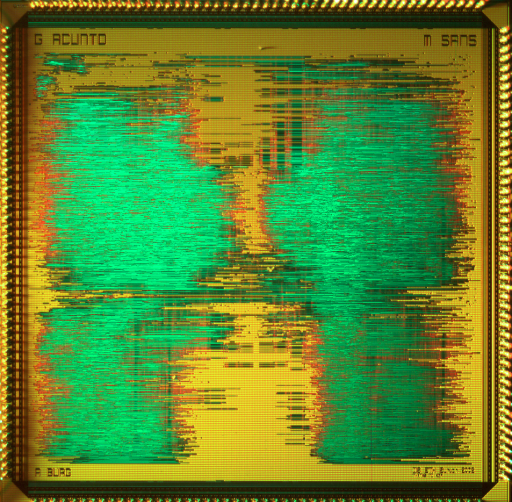# aac (2001)by

### Main Details

 Application Graphics Technology 250 Manufacturer UMC Type Diploma thesis Package PGA208 Dimensions 5000μm x 5000μm Gates 600 kGE Voltage 2.5 V Clock 70 MHz

### Description

Arithmetic coding is a lossless data compression (or entropy coding) scheme. Compared to the more popular prefix-coding schemes (such as Huffman coding) it allows to encode symbols with fractions of a bit and consequently exhibits a much higher compression efficiency. In applications, where the probability distribution of the data source is not a-priori known, or changes over time, an adaptive coding scheme is required. The arithmetic coding algorithm is based directly on the symbol probabilities and does not require an intermediate codebook that needs to be recomputed whenever the distribution changes.

It is therefore very well suited for adaptive implementations. Despite these advantages hardware implementations of the algorithm are rare. The main reasons for this are the facts that an arithmetic coder has a much higher complexity than a prefix en-/decoder and that the original arithmetic coding algorithm uses infinite precision arithmetic. In order to efficiently implement the algorithm with finite precision arithmetic, some minor modifications to the algorithm need to be made.

In this project the core of a configurable arithmetic coder was developed and realized as Virtual Component (VC) in VHDL. It implements the complete en-/decoding algorithm for finite precision arithmetic. Various parameters of the design, such as the number of symbols in the alphabet, the precision of the probabilities, and the resolution of the encoding procedure can be configured. An analysis of the design\u2019s complexity depending on the configuration was performed. For the probability modeler a non-adaptive behavioral model was used. It will be replaced with a real implementation of an adaptive source model in a follow- up project.

### Related Publication

Created by make_cg.pl on Thu Apr 10 09:11:17 2014